Next: Counting experiments Up: Normally distributed observables Previous: Combination of several measurements   Contents

## Measurements close to the edge of the physical region

A case which has essentially no solution in the maximum likelihood approach is when a measurement is performed at the edge of the physical region and the measured value comes out very close to it, or even on the unphysical region. Let us take a numerical example.
Problem:
An experiment is planned to measure the (electron) neutrino mass. The simulations show that the mass resolution is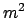eV, largely independent of the mass value, and that the measured mass is normally distributed around the true mass5.4. The mass value which results from the analysis procedure,5.5and corrected for all known systematic effects, iseV. What have we learned about the neutrino mass?
Solution:
Our a priori value of the mass is that it is positive and not too large (otherwise it would already have been measured in other experiments). One can take any vague distribution which assigns a probability density function between 0 and 20 or 30 eV. In fact, if an experiment having a resolution of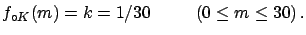eVhas been planned and financed by rational people, with the hope of finding evidence of non-negligible mass, it means that the mass was thought to be in that range. If there is no reason to prefer one of the values in that interval a uniform distribution can be used, for example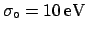(5.21)

Otherwise, if one thinks there is a greater chance of the mass having small rather than high values, a prior which reflects such an assumption could be chosen, for example a half normal with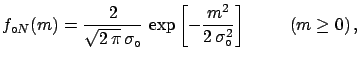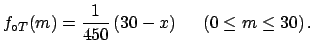(5.22)

or a triangular distribution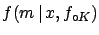(5.23)

Let us consider for simplicity the uniform distribution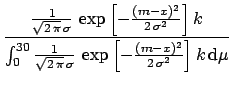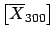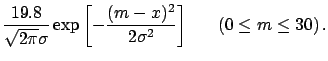(5.24)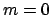(5.25)

The value which has the highest degree of belief is, but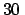is non vanishing up to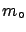eV(even if very small). We can define an interval, starting from, in which we believe that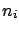should have a certain probability. For example this level of probability can be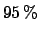. One has to find the value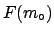for which the cumulative function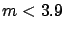equals 0.95. This value ofis called the upper limit (or upper bound). The result is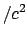eV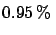at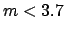probability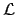(5.26)

If we had assumed the other initial distributions the limit would have been in both cases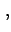eVatprobability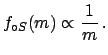(5.27)

practically the same (especially if compared with the experimental resolution ofeV).
Comment:
Let us assume an a priori function sharply peaked at zero and see what happens. For example it could be of the kind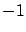(5.28)

To avoid singularities in the integral, let us take a power ofslightly greater than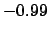, for example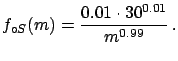, and let us limit its domain to 30, getting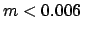(5.29)

The upper limit becomes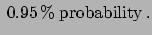eVat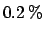(5.30)

Any experienced physicist would find this result ridiculous. The upper limit is less a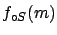of the experimental resolution; rather like expecting to resolve objects having dimensions smaller than a micron with a design ruler! Note instead that in the previous examples the limit was always of the order of magnitude of the experimental resolution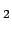. As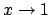becomes more and more peaked at zero (power of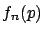) the limit gets smaller and smaller. This means that, asymptotically, the degree of belief thatis so high that whatever you measure you will conclude that: you could use the measurement to calibrate the apparatus! This means that this choice of initial distribution was unreasonable.

Instead, priors motivated by the positive attitude of the researchers are much more stable, and even when the observation is very negative'' the result is stable, and one always gets a limit of the order of the experimental resolution. Anyhow, it is also clear that whenis severalbelow zero one starts to suspect that something is wrong with the experiment'', which formally corresponds to doubts about the likelihood itself.Next: Counting experiments Up: Normally distributed observables Previous: Combination of several measurements   Contents
Giulio D'Agostini 2003-05-15